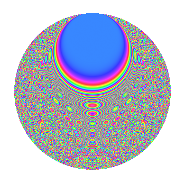# Properties

 Label 8017.2.aLevel 8017 Weight 2 Character orbit a Rep. character $$\chi_{8017}(1,\cdot)$$ Character field $$\Q$$ Dimension 667 Newform subspaces 2 Sturm bound 1336 Trace bound 1

# Related objects

## Defining parameters

 Level: $$N$$ $$=$$ $$8017$$ Weight: $$k$$ $$=$$ $$2$$ Character orbit: $$[\chi]$$ $$=$$ 8017.a (trivial) Character field: $$\Q$$ Newform subspaces: $$2$$ Sturm bound: $$1336$$ Trace bound: $$1$$

## Dimensions

The following table gives the dimensions of various subspaces of $$M_{2}(\Gamma_0(8017))$$.

Total New Old
Modular forms 668 668 0
Cusp forms 667 667 0
Eisenstein series 1 1 0

The following table gives the dimensions of the cuspidal new subspaces with specified eigenvalues for the Atkin-Lehner operators and the Fricke involution.

$$8017$$Dim.
$$+$$$$327$$
$$-$$$$340$$

## Trace form

 $$667q - 3q^{2} - 4q^{3} + 665q^{4} - 2q^{5} - 4q^{6} - 6q^{7} - 15q^{8} + 663q^{9} + O(q^{10})$$ $$667q - 3q^{2} - 4q^{3} + 665q^{4} - 2q^{5} - 4q^{6} - 6q^{7} - 15q^{8} + 663q^{9} - 12q^{10} - 28q^{12} - 8q^{13} - 8q^{14} - 6q^{15} + 657q^{16} - 2q^{17} - 17q^{18} + 2q^{19} - 6q^{20} + 2q^{21} - 36q^{22} - 12q^{23} - 22q^{24} + 643q^{25} - 12q^{26} - 34q^{27} - 38q^{28} - 8q^{29} - 30q^{30} - 6q^{31} - 33q^{32} - 22q^{33} - 14q^{34} + 8q^{35} + 641q^{36} - 26q^{37} - 8q^{38} + 2q^{39} - 26q^{40} + 4q^{41} - 20q^{42} - 12q^{43} - 18q^{44} - 10q^{45} + 18q^{46} - 12q^{47} - 68q^{48} + 661q^{49} - 9q^{50} - 16q^{51} - 38q^{52} - 16q^{53} - 38q^{54} + 10q^{55} - 62q^{56} - 24q^{57} - 52q^{58} + 26q^{59} + 10q^{60} + 8q^{61} - 30q^{62} - 24q^{63} + 609q^{64} - 18q^{65} - 16q^{66} - 30q^{67} + 22q^{68} + 36q^{69} - 80q^{70} + 6q^{71} - 39q^{72} - 28q^{73} - 12q^{74} - 32q^{75} - 22q^{76} + 12q^{77} - 56q^{78} - 6q^{79} - 64q^{80} + 635q^{81} - 44q^{82} - 16q^{83} - 92q^{84} - 8q^{85} - 36q^{86} - 8q^{87} - 144q^{88} + 14q^{89} - 24q^{90} - 26q^{91} - 36q^{92} - 34q^{93} - 44q^{94} - 30q^{95} - 44q^{96} - 40q^{97} - 51q^{98} - 42q^{99} + O(q^{100})$$

## Decomposition of $$S_{2}^{\mathrm{new}}(\Gamma_0(8017))$$ into newform subspaces

Label Dim. $$A$$ Field CM Traces A-L signs $q$-expansion
$$a_2$$ $$a_3$$ $$a_5$$ $$a_7$$ 8017
8017.2.a.a $$327$$ $$64.016$$ None $$-23$$ $$-48$$ $$-55$$ $$-87$$ $$+$$
8017.2.a.b $$340$$ $$64.016$$ None $$20$$ $$44$$ $$53$$ $$81$$ $$-$$

## Hecke characteristic polynomials

There are no characteristic polynomials of Hecke operators in the database